SSC CGL Previous Year Questions: Mensuration - 1

# SSC CGL Previous Year Questions: Mensuration - 1 - SSC CGL

Test Description

## 20 Questions MCQ Test SSC CGL (Tier - 1) - Previous Year Papers (Topic Wise) - SSC CGL Previous Year Questions: Mensuration - 1

SSC CGL Previous Year Questions: Mensuration - 1 for SSC CGL 2023 is part of SSC CGL (Tier - 1) - Previous Year Papers (Topic Wise) preparation. The SSC CGL Previous Year Questions: Mensuration - 1 questions and answers have been prepared according to the SSC CGL exam syllabus.The SSC CGL Previous Year Questions: Mensuration - 1 MCQs are made for SSC CGL 2023 Exam. Find important definitions, questions, notes, meanings, examples, exercises, MCQs and online tests for SSC CGL Previous Year Questions: Mensuration - 1 below.
Solutions of SSC CGL Previous Year Questions: Mensuration - 1 questions in English are available as part of our SSC CGL (Tier - 1) - Previous Year Papers (Topic Wise) for SSC CGL & SSC CGL Previous Year Questions: Mensuration - 1 solutions in Hindi for SSC CGL (Tier - 1) - Previous Year Papers (Topic Wise) course. Download more important topics, notes, lectures and mock test series for SSC CGL Exam by signing up for free. Attempt SSC CGL Previous Year Questions: Mensuration - 1 | 20 questions in 20 minutes | Mock test for SSC CGL preparation | Free important questions MCQ to study SSC CGL (Tier - 1) - Previous Year Papers (Topic Wise) for SSC CGL Exam | Download free PDF with solutions
 1 Crore+ students have signed up on EduRev. Have you?
SSC CGL Previous Year Questions: Mensuration - 1 - Question 1

### The length of one of the diagonals of a rhombus is 48 cm. If the side of the rhombus is 26 cm, then what is the area of the rhombus?    (SSC MTS 2018)

Detailed Solution for SSC CGL Previous Year Questions: Mensuration - 1 - Question 1

Given, Diagonal of a rhombus is 48 cm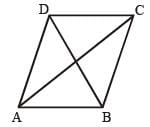Side of rhombus is 26 cm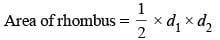The diagonal in a rhombus are perpendicular and bisect of other diagonal.
Given length side 26 cm
One diagonal = 48 cm = DB
Now, OB = OD = 24 cm
Now consider triangle AOB this is a right angled  triangle
and we know AB = 26 cm and OB = 24 cm
Now using the Pythagorus theorem we can find out
length of OA as
OA2 = AB2 – OB2
= 676 – 576
OA2 = 100
OA = 10 cm
So the length of diagonal AC is 2 × 10 = 20 cm.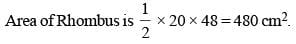SSC CGL Previous Year Questions: Mensuration - 1 - Question 2

### The edge of a cube is 8 cm. What is the total surface area of the cube?    (SSC MTS 2018)

Detailed Solution for SSC CGL Previous Year Questions: Mensuration - 1 - Question 2

Edge of cube = 8 cm
The total surface area of cube is = 6a2
a = 8 cm
S.A. = 6 × 8 × 8 = 64 × 6 = 384 cm2

SSC CGL Previous Year Questions: Mensuration - 1 - Question 3

### What is the circumference of the largest circle which can be inscribed in a square of side 14 cm? (Take π = 22/7)    (SSC MTS 2018)

Detailed Solution for SSC CGL Previous Year Questions: Mensuration - 1 - Question 3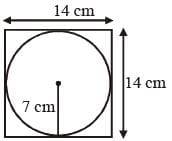Hence the radius of circle is = 7 cm
Circumference of the circle is = 2πr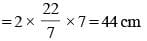SSC CGL Previous Year Questions: Mensuration - 1 - Question 4

Five cubes, each of edge 3 cm are joined end to end. What is the total surface area of the resulting cuboid, in cm2?    (SSC CGL-2018)

Detailed Solution for SSC CGL Previous Year Questions: Mensuration - 1 - Question 4

When we join 5 cubes by end to end
Then length = 5 x 3 = 15 cm
height = 3 cm
total surface Area = 2(15 x 3 + 15 x 3 + 3 x 3) = 198 cm2

SSC CGL Previous Year Questions: Mensuration - 1 - Question 5

The radius of a sphere is reduced by 40%. By what percent will its volume decrease?    (SSC CGL-2018)

Detailed Solution for SSC CGL Previous Year Questions: Mensuration - 1 - Question 5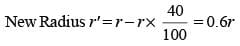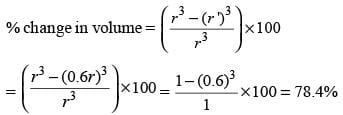SSC CGL Previous Year Questions: Mensuration - 1 - Question 6

A rectangular portion of an airport runway was getting repaired for which an estimate was made on the basis of a rate ₹ R per square unit. But while doing the work, the length of the portion got increased by 10% and the breadth by 8%. Over and above this, there was an increase in the cost of the repair work to the extent of 15%. What was the overall percentage increase in the cost of repair over the estimate?    (SSC CHSL-2018)

Detailed Solution for SSC CGL Previous Year Questions: Mensuration - 1 - Question 6

Let initially length was l and breadth was b.
Area (A) = l × b.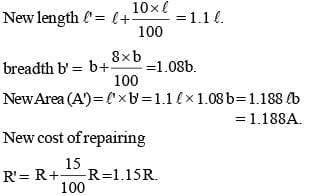Total cost of repairing = 1.15R × 1.188 A = 1.3662 R.A
Percentage increase in cost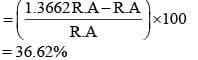SSC CGL Previous Year Questions: Mensuration - 1 - Question 7

The area of a sector of a circle with central angle 60º is A. The circumference of the circle is C. Then A is equal to:    (SSC CHSL-2018)

Detailed Solution for SSC CGL Previous Year Questions: Mensuration - 1 - Question 7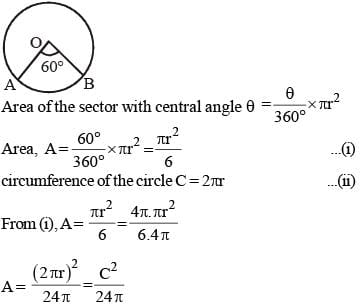SSC CGL Previous Year Questions: Mensuration - 1 - Question 8

The radius of a cylinder is increased by 120% and its height is decreased by 40%. What is the percentage increase in its volume?    (SSC Sub. Ins. 2018)

Detailed Solution for SSC CGL Previous Year Questions: Mensuration - 1 - Question 8

Volume of cylinder (V) = πr2.h, where r = radius h = height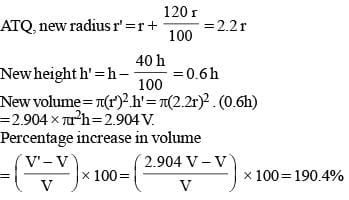SSC CGL Previous Year Questions: Mensuration - 1 - Question 9

One side of a rhombus is 13 cm and one of its diagonals is 24 cm. What is the area of the rhombus?    (SSC Sub. Ins. 2018)

Detailed Solution for SSC CGL Previous Year Questions: Mensuration - 1 - Question 9

We know that diagonal of rhombus bisect perpendicularly. Let ABCD is rhombus and diagonal bisect at point 'O'.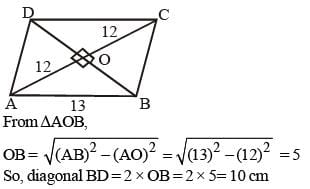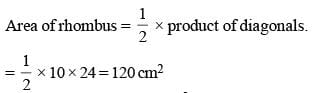SSC CGL Previous Year Questions: Mensuration - 1 - Question 10

The sides of a triangle are 8 cm, 15 cm and 17 cm respectively. At each of its vertices, circles of radius 3.5 cm are drawn. What is the area of the triangle excluding the portion covered by the sectors of the circles (π = 22/7)?    (SSC Sub. Ins. 2018)

Detailed Solution for SSC CGL Previous Year Questions: Mensuration - 1 - Question 10

As, (8)2 + (15)2 = 64 + 225 = 289 = (17)2
Hence, triangle is right angle triangle.
Sum of angle of any triangle is 180°.
Sum of area of all the three sectors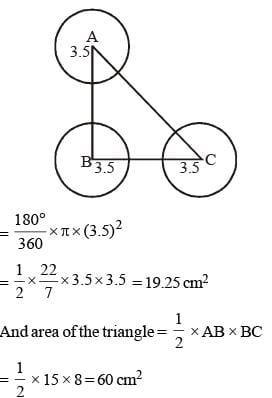So, remaining area = 60 – 19.25 = 40.75 cm2

SSC CGL Previous Year Questions: Mensuration - 1 - Question 11

A sphere of radius 5 cm is melted and recast into spheres of radius 2 cm each. How many such spheres can be made?   (SSC Sub. Ins. 2018)

Detailed Solution for SSC CGL Previous Year Questions: Mensuration - 1 - Question 11

Number of sphere of radius 2 cm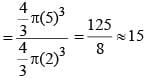SSC CGL Previous Year Questions: Mensuration - 1 - Question 12

6 cubes, each of edge 4 cm, are joined end to end. What is the total surface area of the resulting cuboid?    (SSC Sub. Ins. 2018)

Detailed Solution for SSC CGL Previous Year Questions: Mensuration - 1 - Question 12

After joining 6 cubes of edge length 4 cm each, Length of resulting cuboid = 6 × 4 = 24 cm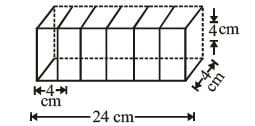Breadth  = 4 cm Height  = 4 cm
Surface Area of the cuboid
= 2(lb +bh + hl)
= 2(24 × 4 + 4 × 4 + 4 × 24)
= 416 cm2

SSC CGL Previous Year Questions: Mensuration - 1 - Question 13

If the perimeter of a rhombus is 80 cm and one of its diagonal is 24 cm, then what is the area (in cm2) of the rhombus?   (SSC Sub. Ins. 2017)

Detailed Solution for SSC CGL Previous Year Questions: Mensuration - 1 - Question 13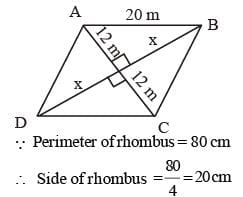By Pythagorean theorem,
(20)2 = (12)2 + (x2)
∴ x2 = 400 – 144 = 256
∴ x = √256 = 16 cm
∴ Diagonal of rhombus = 2x = 2 × 16 = 32 cm and other diagonal = 24 cm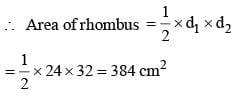SSC CGL Previous Year Questions: Mensuration - 1 - Question 14

A solid cylinder having radius of base as 7 cm and length as 20 cm is bisected from its height to get two identical cylinders.
What will be the percentage increase in the total surface area?    (SSC Sub. Ins. 2017)

Detailed Solution for SSC CGL Previous Year Questions: Mensuration - 1 - Question 14

Here,
Radius of cylinder = 7 cm Height of cylinder = 20 cm
∵ Total surface area  of cylinder = 2πrh + 2πr2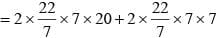= 880 + 308 = 1188 cm2
When cylinder is cuting along height, two new cylinders are generated–radius of new cylinder = 7cm height of new cylinder = 10cm
∵ Total surface area of new cylinder = 2πrh + 2πr2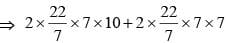⇒ 440 + 308 = 748 cm2
∴ Total surface area of two new cylinders = 748 × 2 = 1496 cm2
∴ Percentage increase in surface area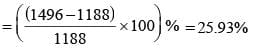SSC CGL Previous Year Questions: Mensuration - 1 - Question 15

Radius of hemisphere is twice that of a sphere. What is the ratio of total surface area of hemisphere and sphere?   (SSC Sub. Ins. 2017)

Detailed Solution for SSC CGL Previous Year Questions: Mensuration - 1 - Question 15

Let radius of hemisphere = 2x
∴ Ratio of total surface area of hemisphere and sphere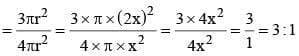SSC CGL Previous Year Questions: Mensuration - 1 - Question 16

The ratio of curved surface area of two cones is 1 : 4 and the ratio of slant height of the two cones is 2 : 1. What is the ratio of the radius of the two cones?    (SSC Sub. Ins. 2017)

Detailed Solution for SSC CGL Previous Year Questions: Mensuration - 1 - Question 16

Here,
The ratio of curved surface area of two cones = 1 : 4
Let curved surface area of first cone be x and curved surface area of second cone be 4x.
Let slant height of first cone be 2x and slant height of second cone be x.
According to question,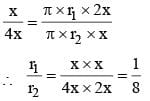∴ The ratio of the radius of the two cones = 1 : 8

SSC CGL Previous Year Questions: Mensuration - 1 - Question 17

If diagonals of a rhombus are 16 cm and 30 cm. then what is the perimeter (in cm) of the rhombus?    (SSC Sub. Ins. 2017)

Detailed Solution for SSC CGL Previous Year Questions: Mensuration - 1 - Question 17

Let side of rhombus be x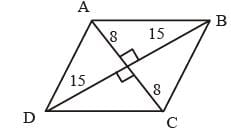By pythagorean theorem,
x2 = (15)2 + (8)2
x2 = 225 + 64 = 289
∴ x = 289= 17 cm
∴ Perimeter of rhombus = 4 × side
= 4 × 17 = 68 cm

SSC CGL Previous Year Questions: Mensuration - 1 - Question 18

A sphere has a total surface area 9 π cm2. Its volume is:   (SSC MTS 2017)

Detailed Solution for SSC CGL Previous Year Questions: Mensuration - 1 - Question 18

Total surface area of sphere = 9π cm2
∵ 4πr2 = 9π cm2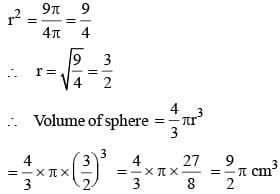SSC CGL Previous Year Questions: Mensuration - 1 - Question 19

The total surface area of a hemisphere is 166.32 sq cm, find its radius?   (SSC CHSL 2017)

Detailed Solution for SSC CGL Previous Year Questions: Mensuration - 1 - Question 19

Here,
Total surface area of hemisphere = 166.32 sq cm.
r = ?
∵ 3πr2 = 166.32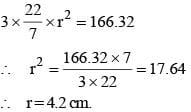SSC CGL Previous Year Questions: Mensuration - 1 - Question 20

If the radius of the cylinder is increased by 25%, then by how much percent the height must be reduced, so that the volume of the cylinder remains same?   (SSC CGL 2017)

Detailed Solution for SSC CGL Previous Year Questions: Mensuration - 1 - Question 20

Volume of the cylinder = πr2h
Since, radius is increased by 25%
Then, increased radius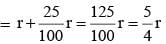Let h1 be the height of cylinder whose radius is increased.
Since, volume remains same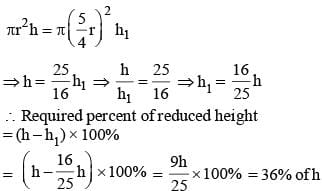So, height must be reduced by 36%.

## SSC CGL (Tier - 1) - Previous Year Papers (Topic Wise)

250 tests
Information about SSC CGL Previous Year Questions: Mensuration - 1 Page
In this test you can find the Exam questions for SSC CGL Previous Year Questions: Mensuration - 1 solved & explained in the simplest way possible. Besides giving Questions and answers for SSC CGL Previous Year Questions: Mensuration - 1, EduRev gives you an ample number of Online tests for practice

250 tests